# ASIDE: Whitworth's Scaling

In his study of the "global gravitational stability [of] one-dimensional polytropes," Whitworth (1981, MNRAS, 195, 967) normalizes (or "references") various derived mathematical expressions for configuration radii, R, and for the pressure exerted by an external bounding medium, Pex, to quantities he refers to as, respectively, Rrf and Prf. The paragraph from his paper in which these two reference quantities are defined is shown here:

In order to map Whitworth's terminology to ours, we note, first, that he uses M0 to represent the spherical configuration's total mass, which we refer to simply as M; and his parameter η is related to our$~n$ via the relation,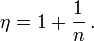$\eta = 1 + \frac{1}{n} \, .$

Hence, Whitworth writes the polytropic equation of state as,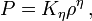$P = K_\eta \rho^\eta \, ,$

whereas, using our standard notation, this same key relation is written as,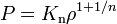$~P = K_\mathrm{n} \rho^{1+1/n}$ ;

and his parameter Kη is identical to our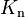$~K_\mathrm{n}$.

According to the second (bottom) expression identified by the red outlined box drawn above,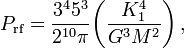$P_\mathrm{rf} = \frac{3^4 5^3}{2^{10} \pi} \biggl( \frac{K_1^4}{G^3 M^2} \biggr) \, ,$

and inverting the expression inside the green outlined box gives,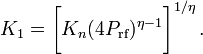$K_1 = \biggl[ K_n (4 P_\mathrm{rf})^{\eta - 1} \biggr]^{1/\eta} \, .$

Hence,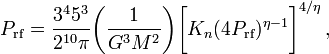$P_\mathrm{rf} = \frac{3^4 5^3}{2^{10} \pi} \biggl( \frac{1}{G^3 M^2} \biggr)\biggl[ K_n (4 P_\mathrm{rf})^{\eta - 1} \biggr]^{4/\eta} \, ,$

or, gathering all factors of Prf to the left-hand side,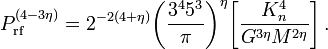$P_\mathrm{rf}^{(4-3\eta)} = 2^{-2(4+\eta)} \biggl( \frac{3^4 5^3}{\pi} \biggr)^\eta \biggl[ \frac{K_n^4}{G^{3\eta} M^{2\eta}} \biggr] \, .$

Analogously, according to the first (top) expression identified inside the red outlined box,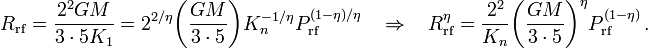$R_\mathrm{rf} = \frac{2^2 GM}{3\cdot 5 K_1} = 2^{2/\eta} \biggl( \frac{GM}{3\cdot 5}\biggr) K_n^{-1/\eta} P_\mathrm{rf}^{(1-\eta)/\eta} ~~~~\Rightarrow~~~~ R_\mathrm{rf}^\eta = \frac{2^{2}}{K_n} \biggl( \frac{GM}{3\cdot 5}\biggr)^\eta P_\mathrm{rf}^{(1-\eta)} \, .$

 Examples$~n$ η = 1 + 1 / n Prf Rrf 1 2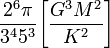$\frac{2^{6}\pi}{3^4 5^3} \biggl[ \frac{G^{3} M^{2} }{K^2}\biggr]$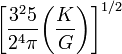$\biggl[ \frac{3^2 5}{2^4 \pi} \biggl( \frac{K}{G} \biggr) \biggr]^{1/2}$ 5 6/5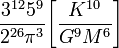$\frac{3^{12} 5^{9}}{2^{26} \pi^3} \biggl[ \frac{K^{10}}{G^9 M^6} \biggr]$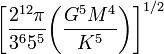$\biggl[ \frac{2^{12} \pi}{3^6 5^5} \biggl( \frac{G^5 M^4}{K^5} \biggr) \biggr]^{1/2}$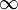$\infty$ 1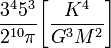$\frac{3^4 5^3}{2^{10}\pi} \biggl[ \frac{K^4}{G^{3} M^{2} }\biggr]$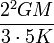$\frac{2^2GM}{3\cdot 5 K}$

 © 2014 - 2021 by Joel E. Tohline |   H_Book Home   |   YouTube   | Appendices: | Equations | Variables | References | Ramblings | Images | myphys.lsu | ADS | Recommended citation:   Tohline, Joel E. (2021), The Structure, Stability, & Dynamics of Self-Gravitating Fluids, a (MediaWiki-based) Vistrails.org publication, https://www.vistrails.org/index.php/User:Tohline/citation